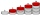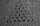# Expression of a variable from the formula + rational numbers - math problems

#### Number of problems found: 7

• Insert 3Insert five arithmetic progression members between -7 and 3/2.
• Positive number zPositive number z is 10% greater than the number y. How many % is y smaller than z? Report the result rounded to one decimal place.
• Average priceSixth grade went on a trip to Moravia. Each of the 26 pupils paid CZK 320 and the school paid a total of CZK 3,510. What was the average price of a trip per student?
• Sea waterMixing 62 kg of sea water with 84 kg rainwater is created water containing 3.1% salt. How many percent sea water contains salt?
• Rectangular cuboidThe rectangular cuboid has a surface area 5334 cm2, and its dimensions are in the ratio 2:4:5. Find the volume of this rectangular cuboid.
• Two carsTwo cars started against each other at the same time to journey long 346 km. The first car went 53 km/h and the second 53 km/h. What distance will be between these cars 20 minutes before meet?
• BoatsThree-quarters of boats are white, 1/7 are blue and 9 are red. How many boats do we have?

We apologize, but in this category are not a lot of examples.
Do you have an interesting mathematical word problem that you can't solve it? Submit a math problem, and we can try to solve it.

We will send a solution to your e-mail address. Solved examples are also published here. Please enter the e-mail correctly and check whether you don't have a full mailbox.

Please do not submit problems from current active competitions such as Mathematical Olympiad, correspondence seminars etc...
Expression of a variable from the formula - math problems. Rational numbers - math problems.